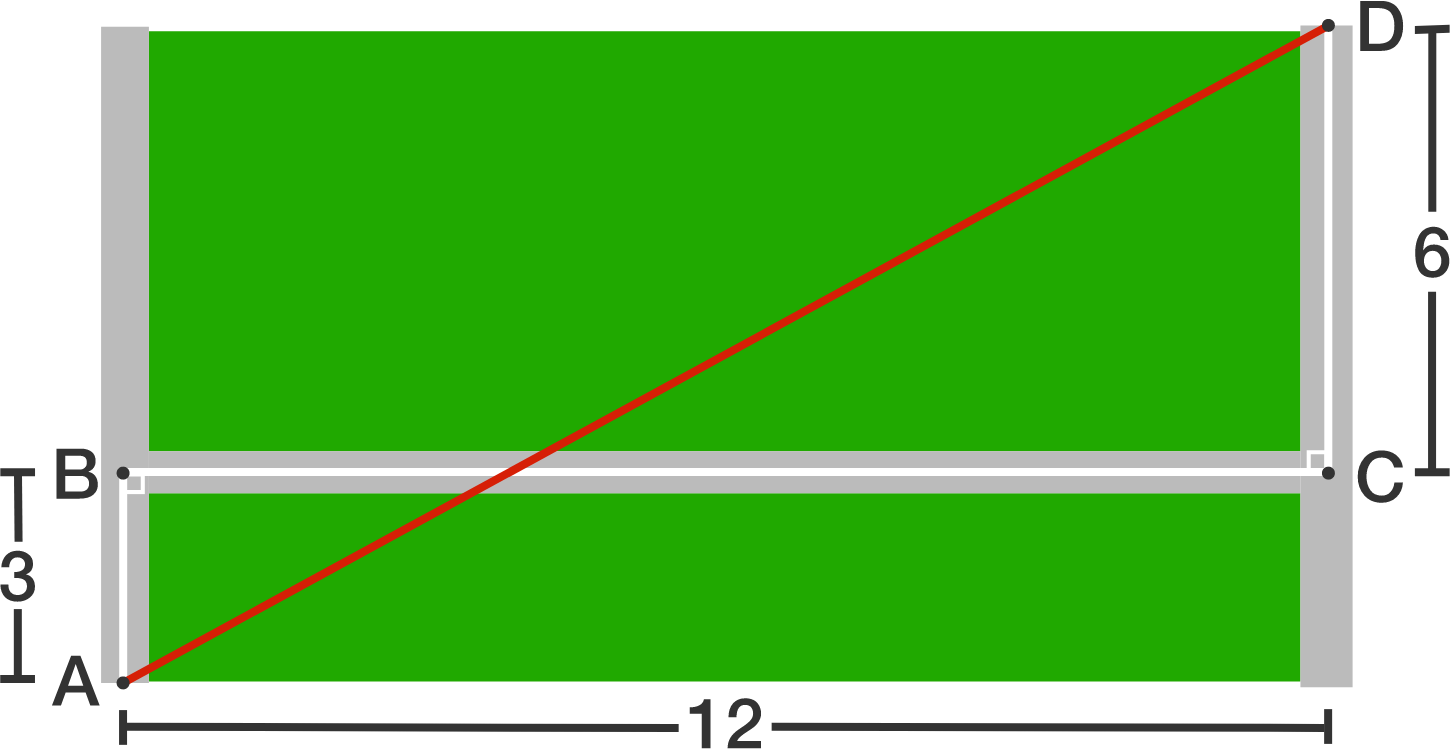# Keep Off The Grass

Geometry Level 1The diagram above shows a big rectangular lawn cut into two smaller rectangles, by the line $BC$, with dimensions $3 \times 12$ and $6 \times 12$ each. What is the length of $AD?$

×